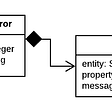# NumPy a Boon Package!!!

NumPy (Numerical Python) is an open source Python library ,a fundamental package for scientific computing in Python. Some of the features we’ll find in NumPy :

1. It is a multidimensional array object, providing fast array-oriented arithmetic operations and efficiently broadcast operation across dimensions.
2. Tools for reading/writing array data to disk and working with memory-mapped files, It is designed for efficiency on large array of data.
3. Provide implementations of many functions across linear algebra, statistics.
4. NumPy operations perform complex computations on entire arrays without the need for Pythons for loops.

## Comparing performance :

Consider a NumPy array of one million integers, and the equivalent Python list :

NumPy based algorithms are generally 10 to 100 times faster (or more) than Python list and use significantly less memory.

# The NumPy ndarray: A Multidimensional Array object

Creating ndarray :

`arr = np.array([[1,1,2,3],[5,8,13,21]])arr>> array([[ 1,  1,  2,  3],           [ 5,  8, 13, 21]])arr.shape #shape attribute gives size of array along each dimension>> (2,4)arr.ndim   #ndim attribute gives the dimensions of an array>> 2This array has 2 axes. First axis has a length of 2 and the second axis has a length of 4.Note : In NumPy, dimensions are called axes.`

# NumPy Operations

we can perform arithmetic operations such as add(), subtract(), multiply(), and divide() in a ndarray(n-dimensional array) must be either of the same shape or should confirm to array broadcasting rules. Few operations of NumPy are shown below :

`'Adding the two arrays a and b' : >>  np.add(a,b) or a+b'Subtracting the two arrays a and b' : >>  np.subtract(a,b) or a-b'Element-wise multiplication of a and b': >> np.multiply(a,b) or a*b'Matrix wise multiplication of a and b' : >> np.dot(a,b)'Exponential of all elements in a': >> np.exp(a)'Sine function of all element in a': >> np.sin(a)'Squareroot of all element in a' : >> np.sqrt(a)'Zeros Matrix of size (a x b)' : >>  np.zeros((a, b))`

It describe how numpy treats with the different shapes during arithmetic operation. Smaller array is “broadcast” across the larger array so that they have compatible shapes.

# Stats with NumPy

NumPy has quite a few useful statistical functions for finding minimum, maximum, percentile , standard deviation and variance, etc. of the given elements in the array.

`np.amin(a) >>Return the minimum of an array or minimum along an axisnp.amax(a) >>Return the maximum of an array or maximum along an axisnp.mean(a) >>Compute the arithmetic mean along the specified axisnp.std(a) >>Compute the standard deviation along the specified axis`

--

--

--

## More from Nerd For Tech

NFT is an Educational Media House. Our mission is to bring the invaluable knowledge and experiences of experts from all over the world to the novice. To know more about us, visit https://www.nerdfortech.org/.

## Developing on a Virtual Machine from your local IDE## How to connect to Mainframe from your Mac laptop using x3270## Cross-chain Bridges## Secure your Secrets with the power of “git-crypt”## Abstraction and Encapsulation: Python OOP Complete Course — Part 8## 7 Reasons to Migrate to Salesforce Lightning Before Winter 2019## Testing a Spring Boot RESTful Service## Abu Qais

The price of “anything” is the amount of “time”, U xchange for it. Education | Technology | Data Science | Statistics | History

## Fix Attribute Error and Runtime Error in tensorflow and keras | Beginner Must Read## Python-P01-Teach Me Python !## 3 Disturbing Consequences of Using Data Unethically